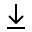MathBin Line Member

A line is a one-dimensional object that exists in one dimension or higher. A line has infinite length, zero width, and zero height. Two (or more) points define a line. The following equation gives a non-vertical line in two-dimensions where m is the slope (gradient) of the line, b is the y-intercept of the line, and x is the independent variable of the function y = f(x).

y = m x + b

In two dimensions, the following equation describes every line L (including vertical lines), where a, b, and c are real coefficients. Note that the line is vertical when b is zero, and the line is horizontal when a is zero.

a x + b y = c, where a and b are not both zero

In three dimensions, a line is given by the following two linear equations, where (a1, b1, c1) and (a2, b2, c2) are not proportional. This follows since a single linear equation describes a plane and two distinct planes intersect at a line.

a1 x + b1 y + c1 zd1 = 0
a2 x + b2 y + c2 zd2 = 0
Bins in Two Dimensions